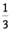## OBJECTIVE: To learn how to change the standard form of the equation of a line to slope-intercept form

In previous lessons, you learned how to graph lines written in various forms. Here, you are going to learn how to change the STANDARD FORM of the equation of a line to SLOPE-INTERCEPT FORM. The applet below demonstrates the process. Repeat as many times as needed to master the concept.
Here are the steps: 1. Begin isolating y by subtracting x-values from both sides of the equation. 2. Divide both sides of the resulting equation by the coefficient of y. 3. Reduce fractions to lowest terms. Use the applet below for practice. In the upper left-hand corner of the applet, you'll see the STANDARD FORM (Ax + By = C) of the equation of a line. Your job is to rewrite this equation into SLOPE-INTERCEPT FORM (y = mx + b). When entering ratios/fractions, be sure to enter EXACT VALUES. (Example: Enter "1/3" forand NOT 0.33). If you type in a correct equation, you'll see a big "CORRECT !!!" sign appear. Otherwise, you'll have to try again. Repeat as many times as needed to master the concept.

## TODAY you learned how to change the STANDARD FORM of the equation of a line into SLOPE-INTERCEPT FORM.

In future lessons, you'll learn how to derive the equation of a line given different conditions. Did you ENJOY doing the exercises?